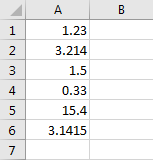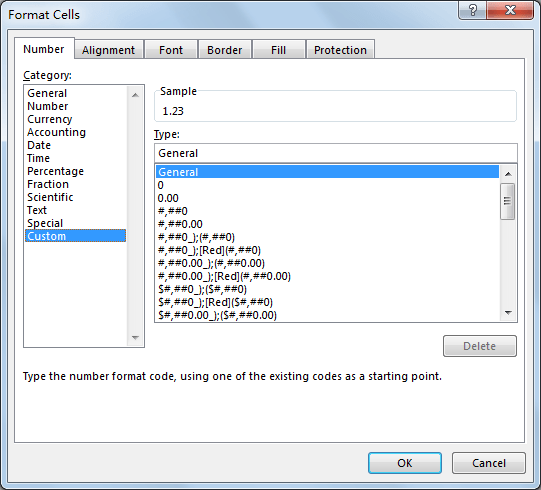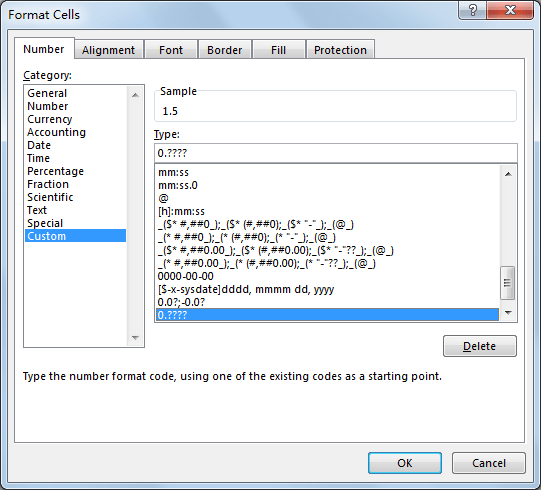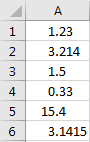# How to Align Column of Numbers by Decimal Point in Excel

When entering a column of numbers with decimal point, these numbers are aligned right or left by default setting, anyway, they are not aligned by decimal point. If we want to make these numbers aligned by decimal point to make them neatly, we can via Format Cells to customize type of number, then these numbers will be aligned by decimal point properly, this article will show you the details step by step.

Precondition:

First, we need to create a list of numbers. Make sure that all numbers contain decimal point.You can see that above numbers are aligned right by default. In excel Home->Alignment group, you can align text right, left or center, but you cannot align numbers by decimal point.

## Align Numbers in A Column by Decimal Point in Excel

Step 1: Select the list of numbers, then right click to load menu. Click Format Cells on menu.Step 2: On Format Cells, under Number tab, click Custom.Step 3: Under Type enter 0.????. Then click OK.Step 4: Verify that numbers are aligned by decimal point properly.1. ?’ in ‘0.????’ represents the decimal digit, if you have numbers like 0.23 with only two digits after decimal point, you can enter 0.?? Instead of 0.????. You can enter only one ? or multiple ? per your demands.
2. If you want to align numbers by decimal point, but keep only two decimal digits, you can also enter ‘0.??‘ under Type.
3. You can also enter other type here to align numbers by decimal point. For example, “_-???0.0??;-???0.0??;” or “0.0?;-0.0?”.

Related Posts

Cap percentage values between 0 and 100

This article will talk about how to cap the percentage values between 0% and 100% in Microsoft Excel Spreadsheet or Google Sheets. If you are a newbie on Excel or google Sheets, you may be able to do this by ...

Calculate Cap Percentages to Specific Value

This article will talk about how to limit the cap percentage of a given amount to a given value in Microsoft Excel Spreadsheet or Google Sheets. If you are a newbie on Excel or google Sheets, you may be able ...

Calculate Win Loss Tie

Suppose you got a task to calculate the win, loss, and tie totals; what would you do? If you are new to Ms Excel and don't have enough experience with it, then you might do this task manually but let ...

Calculate Years Between Dates In Ms Excel

If you are an avid Ms Excel user, then you might have come across a task in which you needed to calculate the years between the dates; you might take it easy and do this task manually, which is also ...

Calculate Number of Hours between Two Times

Calculating the difference between two times might be a valuable statistic for subsequent computations or averages, whether you're producing a time sheet for staff or recording personal exercises. While Excel has a plethora of complex functions, including date and time ...

Calculate Loan Interest in Given Year

When you borrow money, you are supposed to repay it gradually. Lenders, on the other hand, want to be compensated for their services and the risk they incur by lending you money. That is, you will not just repay the ...

Calculate Interest Rate for Loan

The interest rate is the fee charged by a lender to a borrower and is expressed as a percentage of the principal—the lent amount. The interest rate on a loan is often expressed as an annual percentage rate, abbreviated as ...

Calculate Interest for Given Period

Using the IPMT function in Excel, we can compute the interest payment on any loan. This step-by-step tutorial will guide Excel users of all skill levels through the process to calculate interest for given period. Finally, the formula: =IPMT(B3/12,1,B5,-B2) The ...

How To Use Excel GCD Function

This post will guide you how to use Excel GCD function with syntax and examples in Microsoft excel. Description The Excel GCD function Returns the greatest common divisor of two or more integers. So you can use the GCD function ...

Calculate A Ratio From Two Numbers In Excel

In elementary mathematics, a ratio is a connection or comparison between two or more integers. For example, ratios are often expressed as ":" to demonstrate the relationship between two numbers. You would think that manually calculating a ratio from two ...

Sidebar